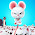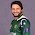In this post, I am going to provide you a list of some books which are not only standard but also recommended by many scholars.

## General Physics

1. Fundamentals of Physics: David Halliday, Jearl Walker, and Robert Resnick
2. University Physics : Roger A. Freedman

## Classical Mechanics

1. Herbert Goldstein: Classical Mechanics
2. Introductory: The Feynman Lectures, vol 1.
3. Keith Symon: Mechanics
4. V.I. Arnold: Mathematical methods of classical mechanics
5. Marion & Thornton: Classical Dynamics of Particles and Systems
6. John R. Taylor: Classical Mechanics

## Classical Electromagnetism

1. Jackson: Classical Electrodynamics, 2nd ed.
2. Griffiths: Inroduction to Electrodynamics
3. Purcell: Berkeley Physics Series Vol 2
4. Reitz, Milford and Christy: Foundations of Electromagnetic Theory
5. Feynman: The Feynman Lectures, Vol. 2
6. Lorrain & Corson: Electromagnetism, Principles and Applications
7. Same level as Jackson and with lots of material not in Jackson.
8. Marion and Heald: Classical Electromagnetic Radiation

## Quantum Mechanics

1. Cohen-Tannoudji: Quantum Mechanics I & II&
2. Sakurai: Modern Quantum Mechanics
4. Griffiths: Introduction to Quantum
5. Shankar: Principles of Quantum mechanics
6. H. Everett: Theory of the Universal Wavefunction
7. Bjorken and Drell: Relativistic Quantum Mechanics/ Relativistic Quantum Fields
8. Messiah : Quantum Mechanics
9. Friedman and Leonard Susskind: Quantum mechanics the theoretical minimum
10. Dirac: Principles of Quantum Mechanics
11. Feynman: The Feynman Lectures, Vol. 3
12. John von Neumann: Mathematical foundations of quantum mechanics
13. Schiff: Quantum Mechanics
14. Eisberg and Resnick: Quantum Physics of Atoms, Molecules, Solids, Nuclei, and Particles

## Statistical Mechanics

1. David Chandler: Introduction to Modern Statistical Mechanics, 1987
2. R. Tolman: Principles of Statistical Mechanics. Dover
3. Kittel & Kroemer: Statistical Thermodynamics
4. Reif: Principles of statistical and thermal physics.
5. Felix Bloch: Fundamentals of Statistical Mechanics.
6. Thermodynamics, by H. Callen.
7. Statistical Mechanics, by R. K. Pathria
8. Shang keng Ma: Statistical Mechanics

## Condensed Matter

1. Charles Kittel: Introduction to Solid State Physics (ISSP),
2. Ashcroft and Mermin: Solid State Physics,
3. Charles Kittel: Quantum Theory of Solids.
4. Solid State Theory, by W. A. HarrisonTheory of Solids, by Ziman.
5. Marvin L. Cohen and Steven Gwon Sheng Louie: Fundamental of Condensed matter Physics
6. Paul Chaikin: Principles of condensed matter physics

## Special Relativity

1. Taylor and Wheeler: Spacetime Physics Still the best introduction out there.
2. Relativity: Einstein's popular exposition.
3. Wolfgang Rindler: Essential RelativityA.P. French: Special Relativity
4. Special Relativity and its Experimental Foundations Yuan Zhong Zhang
5. Robert Resnick: Introduction to Special relativity
6. Einstein: Relativity: The Special and the General Theory

## Particle Physics

1. Kerson Huang: Quarks, leptons & gauge fields, World Scientific
2. L. B. Okun: Leptons and quarks, translated from Russian by V. I. Kisin, North-Holland, 1982.
3. T. D. Lee: Particle physics and introduction to field theory.
4. Itzykson: Particle Physics
5. Bjorken & Drell: Relativistic Quantum MechanicsFrancis Halzen & Alan D. Martin: Quarks & Leptons,
6. Donald H. Perkins: Introduction to high energy physics
7. Mandl, Shaw: Quantum Field Theory
8. F.Gross: Relativistic Quantum Mechanics and Field Theory
9. S. Weinberg: The Quantum Theory of Fields, Vol I,II, 1995
10. M. Kaku: Strings, Conformal Fields and Topology
11. R.P. Crease, C.C. Mann: The Second Creation 1996
12. Palash b. pal: An introductory course of particle physics

## General Relativity

1. Robert M. Wald: Space, Time, and Gravity: the Theory of the Big Bang and Black Holes.
2. Schutz: A First Course in General Relativity.
3. Weinberg: Gravitation and Cosmology
4. Hans Ohanian: Gravitation & Spacetime
5. Charles W. Misner and Kip Thorne: Gravitation
6. Robert Wald: General Relativity
7. Clifford Will: Was Einstein Right?.
8. Kip Thorne: Black Holes and Time Warps: Einstein's Outrageous Legacy
9. Carroll: Spacetime and Geometry: An Introduction to General Relativity

## Mathematical Methods

1. Morse and Feshbach: Methods of Theoretical Physics.
2. Mathews and Walker: Mathematical Methods of Physics.
3. Arfken: Mathematical Methods for Physicists Academic Press
4. Zwillinger: Handbook of Differential Equations. Academic Press
5. F.W. Byron and R. Fuller: Mathematics of Classical and Quantum Physics (2 vols)
6. Robert Hermann: Lie groups for physicists, Benjamin-Cummings, 1966.
7. George Mackey: Unitary group representations in physics, probability, and number theory.
8. Charles Nash and S. Sen: Topology and geometry for physicists.
9. Bamberg and S. Sternberg: A Course of Mathematics for Students of Physics
10. Bishop & Goldberg: Tensor Analysis on Manifolds.
11. Dodson & Poston: Tensor Geometry.
12. Abraham, Marsden & Ratiu: Manifolds, Tensor Analysis and Applications.
13. M. Nakahara: Topology, Geometry and Physics.
14. Courant and Hilbert: Methods of Mathematical Physics Wiley

## Nuclear Physics

1. Preston and Bhaduri: Structure of the Nucleus
2. Blatt and Weisskopf: Theoretical Nuclear Physics
3. Satchler: Direct Nuclear Reactions
4. Krane: Introductory nuclear physics

## Cosmology

1. J. V. Narlikar: Introduction to Cosmology.1983 Jones & Bartlett Publ.
2. Hawking: A Brief History of Time
3. Weinberg: First Three Minutes
4. Timothy Ferris: Coming of Age in the Milky Way and The Whole Shebang
5. Kolb and Turner: The Early Universe.
6. Black Holes and Warped Spacetime, by William J. Kaufmann III.
7. M.V. Berry: Principles of Cosmology and Gravitation
8. T. Padmanabhan: Structure formation in the universe
9. P.J.E. Peebles: The large-scale structure of the universe

A physics Book ListReviewed by Unknown on 01:11 Rating: 5

1.2.3.